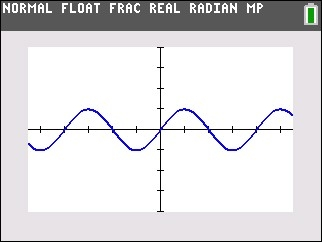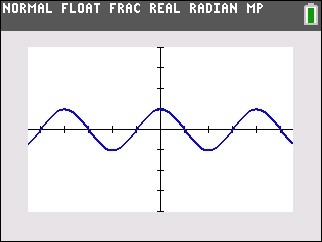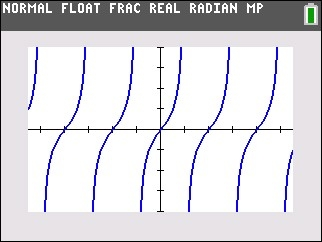# Knowledge Base

## Solution 34673: Graphing Sine, Cosine, and Tangent Functions on the TI-83 Plus and TI-84 Plus Family of Graphing Calculators.

### How do I graph sine, cosine, and tangent functions on the TI-83 Plus and TI-84 Plus family of graphing calculators?

The following examples will demonstrate how to graph sine, cosine and tangent functions on the TI-83 Plus and TI-84 Plus family of graphing calculators. Each device has the ability to graph trigonometric function in either degrees or radians.

Please Note: The example functions below use Radian mode. Radian mode is recommended when graphing a trigonometric and an algebraic function on the same screen. Degree Mode can be used as well but may not match the images below until the fourth step to use ZOOM Ztrig is used.

1) Press the [MODE] key.
2) Highlight Radian and press the [ENTER] key.

Example 1: Graph Sin(X)

1) Press the [Y=] key to access the Y= Editor.
2) With the cursor next to Y1, press [SIN] [X,T,q,n] [ )].
3) Press the [GRAPH] key to graph the function.
4) To display a better graph of the sin(x) function, press [ZOOM] and select 7:ZTrig.Example 2: Graph Cos(X)

1) Press the [Y=] key to access the Y= Editor.
2) With the cursor next to Y1, press [COS] [X,T,q,n] [ )].
3) Press the [GRAPH] key to graph the function.
4) To display a better graph of the cos(x) function, press [ZOOM] and select 7:ZTrig.Example 3: Graph Tan (X)

1) Press the [Y=] key to access the Y= Editor.
2) With the cursor next to Y1, press [TAN] [X,T,q,n] [ )].
3) Press the [GRAPH] key to graph the function.
4) To display a better graph of the tan(x) function, press [ZOOM] and select 7:ZTrig.Please see the TI-83 Plus and TI-84 Plus Family guidebooks for additional information.